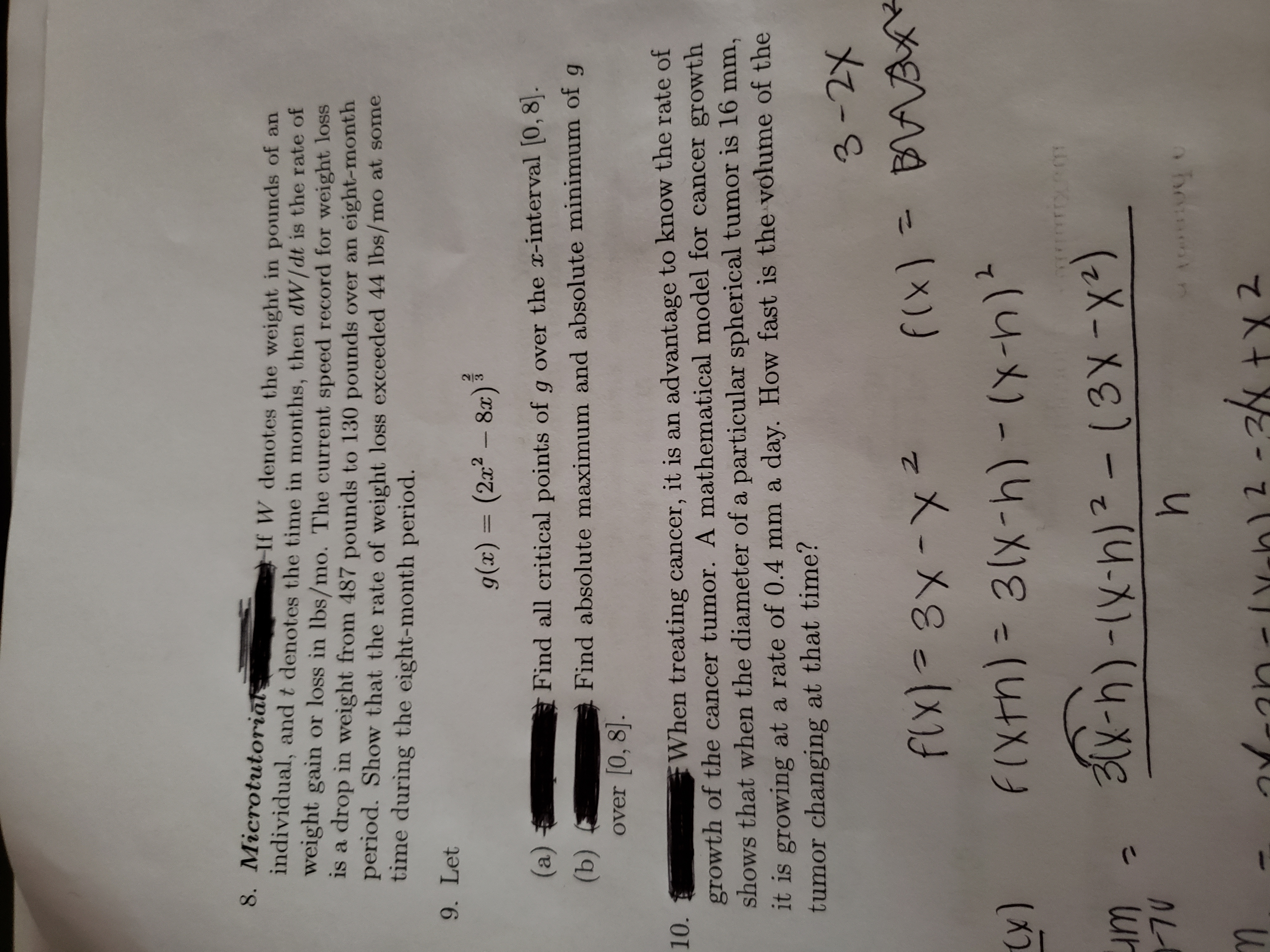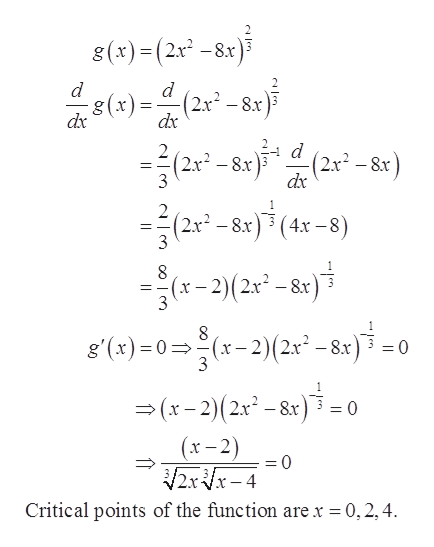# 8. MicrotutoriātIf W denotes the weight in pounds of anindividual, and t denotes the time in months, then dW / dt is the rate ofweight gain or loss in lbs/mo. The current speed record for weight lossis a drop in weight from 487 pounds to 130 pounds over an eight-monthperiod. Show that the rate of weight loss exceeded 44 lbs/mo at sometime during the eight-month period.9. Letg(x) = (2x² – 8a)³%3D(a)Find all critical points of g over the x-interval (0,8].(b)over 0, 8].Find absolute maximum and absolute minimum of gWhen treating cancer, it is an advantage to know the rate ofgrowth of the cancer tumor. A mathematical model for cancer growthshows that when the diameter of a particular spherical tumor is 16 mm,10.it is growing at a rate of 0.4 mm a day. How fast is the volume of thetumor changing at that time?3-2XAXYf(x) = BVABflx)=3x-x2F(xtn) = 3(x-h) -(x-)*(x)(3X-X2)214-X)-(31x2/kYran-lY-h)2 -36 +x 2

Question
9 views

#9help_outlineImage Transcriptionclose8. Microtutoriāt If W denotes the weight in pounds of an individual, and t denotes the time in months, then dW / dt is the rate of weight gain or loss in lbs/mo. The current speed record for weight loss is a drop in weight from 487 pounds to 130 pounds over an eight-month period. Show that the rate of weight loss exceeded 44 lbs/mo at some time during the eight-month period. 9. Let g(x) = (2x² – 8a)³ %3D (a) Find all critical points of g over the x-interval (0,8]. (b) over 0, 8]. Find absolute maximum and absolute minimum of g When treating cancer, it is an advantage to know the rate of growth of the cancer tumor. A mathematical model for cancer growth shows that when the diameter of a particular spherical tumor is 16 mm, 10. it is growing at a rate of 0.4 mm a day. How fast is the volume of the tumor changing at that time? 3-2X AXY f(x) = BVAB flx)=3x-x2 F(xtn) = 3(x-h) -(x-)* (x) (3X-X2) 214-X)-( 31x 2/k Yran-lY-h)2 -36 +x 2 fullscreen
check_circle

Step 1

To determine:

Part a) The critical points on the interval [0, 8].

Part b) Absolute maximum and minimum on the interval [0, 8].

Step 2

Given:

Step 3

Part a)

Compute critical points of ...help_outlineImage Transcriptionclose8(x)=(2x² -8x) 8(*) = (2* - 8r)5 -(2.x² – 8x) dx dx -(2x² – &r) dx (2x² – 8x 3 - 8x (2r - 8x)*(4x -8) (2x* – &r)*(4. (x– 2)(2x² – &r) 3 g'(x)=0=(x- 2)(2r² - 8r) =(x-2)(2r² - &x) = (x– 2) %3D 2x Vx- 4 Critical points of the function are x = 0,2,4. fullscreen

### Want to see the full answer?

See Solution

#### Want to see this answer and more?

Solutions are written by subject experts who are available 24/7. Questions are typically answered within 1 hour.*

See Solution
*Response times may vary by subject and question.
Tagged in
MathCalculus

### Functions